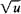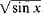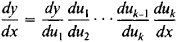# Composite Function

Also found in: Dictionary, Wikipedia.

## composite function

[kəm′päz·ət ′fəŋk·shən]
(mathematics)
A function of one or more independent variables that are themselves functions of one or more other independent variables.
McGraw-Hill Dictionary of Scientific & Technical Terms, 6E, Copyright © 2003 by The McGraw-Hill Companies, Inc.
The following article is from The Great Soviet Encyclopedia (1979). It might be outdated or ideologically biased.

## Composite Function

a function of a function. Let us suppose that the variable y is a function of u, that is, y = f(u), and that u is in turn a function of x, that is, u = Φ(x). Then y is a composite function of u and x—that is, y = f[Φ(x)]—defined for all x such that Φ(x) is in the domain of f(u). In this case, y is said to be a composite function of the variable x and the variable u, which is sometimes called the intermediate variable. For example, if y = u2 and u = sin x, then y = sin2x for all values of x. If, however,and u = sin x, then, which, if we are restricted to real values of the function, is defined only for all x such that sin x ≥ 0—that is, for 2kπx ≥ (2k + 1)π, where k = 0, ± 1, ± 2,….

The derivative of a composite function is equal to the product of the derivative of the function with respect to the intermediate variable and the derivative of the intermediate variable with respect to the independent variable. This rule, called the chain rule, extends to composite functions with two, three, or more intermediate variables. Thus, if y = f(u1, u1 = Φ(u2), …, uk–1 = Φk–1(uk), uk = Φk(x), thenMentioned in ?
References in periodicals archive ?
The Structure Method of the Total Derivative of Several Variables Composite Functions
in [9, 10] to propose a structure of the composite function wavelet neural networks (CFWNN) to be used with ELM for different applications.
The proposition is the extension of the theorem from  and it shows how the partial derivative of the total delta derivative of the composite function with a vector argument can be expressed through the total delta derivative of the partial derivative of the composite function.
[G.sub.1](y(t)) and [G.sub.2](y(t)) are composite functions or nonlinear functions.
Chen: An explicit formula of Hessian determinants of composite functions and its applications, Kragujevac J.
It is a composite function. I have realised that I have been applying the chain rule when I wrote u.
The CL-930 has a composite function of guiding and expansion and is made of a web-expanding roller, a web guide sensor and a control panel.
For the proof a limit theorem for composite function F([L.bar](s, [[chi].bar]], [[alpha].bar], [a.bar])), we will apply an assertion on the preservation of the weak convergence under mappings.
The deduction of the necessary conditions involves frequent application of the differentiation of the composite functions with respect to time argument and taking the partial derivatives of the differentiated composite function with respect to one of the variables or its derivatives.
Given the functions F: A [right arrow] [R.sup.m] and g: [R.sup.m] [right arrow] R, consider the composite function g [omicron] F: A [right arrow] R such that the following conditions are fulfilled:
As x = x[q([alpha])] that by a rule of differentiation of the composite function, assuming that rank-[partial derivative]q/[partial derivative][[alpha].sup.T] = m and matrix [partial derivative]q/[partial derivative][[alpha].sup.T] is non-singular, we will receive: [MATHEMATICAL EXPRESSION NOT REPRODUCIBLE IN ASCII].

Site: Follow: Share:
Open / Close